Kart Speed Short Version

How to Find YourGo-Kart Speed Without Using a Speedometer

Printed with permission on the “Complete Kart Builders Web Site”

Update: Dale Thomas has recently got in touch, and has provided an Excel file which can be used to do all the calculating for you. As seen in the image below, all you need to enter is:
1. Number of Teeth on the Sprocket on the Engine
2. Number of Teeth on the Sprocket on the Axle
3. Diameter of Rear Wheel (in inches)
4. RPM of the Engine (usually printed on a sticker on the engine)
Feel free to contact Dale at samohtelad*nospamatall*@aol.com (removing the *nospamatall*).

You can find your go kart speed without using a speedometer.  There are at least two ways to find the speed.  I’ll call the ways Method 1 and Method 2.  Method 1 doesn’t require a tachometer, but you will need a cheap calculator, a watch with a second hand and a known, accurately pre-measured distance (2 to 4 km or 1 to 2 miles) where you safely can ride your kart at full speed.  For Method 2 you’ll need a cheap calculator, a measuring device, pen & paper and it requires using a tachometer.  Please keep safety in mind at all times for you, for passersby, for the property of others and for the kart.  Also, perform all measuring and counting steps with the engine completely stopped.

There are some formulas to use, but they’re pretty simple.   I’ll show how to do the speed calculations for the metric and for the American measurement system.  Each step used will be introduced in a logical order and a description of each step will be given.  I’m giving a bare bones account of each method.  I have a detailed set of steps outlined in an article that runs 10 pages.  Some of the instructions are somewhat repetitive, but the article is aimed at teaching concepts rather than just as a presentation of a set of formulas to follow. Many of you will find the bare bones presentation enough of a description to get the job done.   If there are those reading this who desires the full article I’ll be happy to email it to you from carhouse1nospanatall@hotmail .com.  If you copy and paste the email address you must delete the nospamatall part of the address.  If you do not delete that phrase I’ll never get your email.  Following are the steps to find your kart speed without using a speedometer.

Method 1

Method 1 requires you to ride your kart at full speed over an accurately pre-measured distance and to keep track of the exact amount of time it takes.   First, instructions are for the metric system then immediately following are instructions for the American version.

KPH

To find the KPH of your kart, say for example that you rode 4 km and it took exactly 8 minutes.  You need the distance in meters and the time in seconds.  That’s 4000 meters and 480 seconds.  The formula is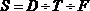where S is speed in kph, D is the distance in meters, T is time in seconds and F is 0.27778.   F is a conversion factor.  The factor was found by dividing 1000 meters by 3600, the number of seconds in an hour.  It means that one kilometer per hour is equal to 0.27778 of a meter per second.  Substituting in the formula we have.  And the speed, S, is 29.999 KPH.

MPH

To find the MPH of your kart, say for example that you rode 2-1/2 miles and it took 8 minutes.  You need the distance in feet and the time in seconds.  Multiply 2-1/2 miles by 5280 and get 13,200 feet and then multiply 8 minutes by 60 seconds to get 480 seconds. The formula iswhere S is speed in mph, D is the distance in feet, T is time in seconds and F is 1.46667.  The conversion factor is different this time because we’re using the American measuring system.  It was found by dividing 5280 (the number of feet in a mile) by 3600, the number of seconds in an hour.  It means that one mph is equal to 1.46667 feet per second.  Substituting in the formula we have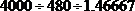.  And, S, the speed is 18.75 mph.

Method 2

Method 2 takes a bit longer to calculate, is a bit more complicated, requires the use of a tachometer and needs information you will get directly from your kart.  Method 2 also uses more formulas but each one is about as simple and as easy as the one above.  The formulas will be worked in a logical sequence of steps.  We’ll figure the mph first then afterwards we’ll figure the kph.

MPH

We are going to follow a very logical set of steps to find your kart speed consisting of finding the following: 1) the distance your drive wheel moves the kart while rotating only once, 2) how far the kart moves in a minute by multiplying the distance it moves in one revolution by the number if times the wheel rotates in each minute, and 3) the speed per hour by converting from the distance per minute.

First get the information you need from your kart.  Before riding the kart and with the engine turned off, measure exactly, the outside diameter of your drive tire, and then count the number of sprocket teeth on the engine clutch and the number of teeth on the axle sprocket, making certain to count each sprocket tooth only once.  You also need to measure the maximum rpm of your engine while riding the kart at full speed over a level surface.

Let’s say for example that your drive tire measured 15 inched in diameter, that the engine clutch sprocket has 10 teeth, the axle sprocket has 60 teeth and that your maximum engine rpm while riding the kart is about 3000.

Step 1 is finding the distance your drive tire moves the kart moves in only rotation.  The circumference of the drive tire is that distance.  The circumference is found with the following formula,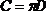where C is the drive tire circumference in feet,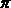is 3.1416 and D is the drive tire outside diameter.  Substituting in the formula we havewhich gives C= 47.124 inches.  We’ll need measurements in feet for this calculation, so change the circumference, found in inches, into feet by dividing the inches by 12.  This gives the drive tire circumference, and the distance the kart will move with only one drive tire rotation equals 3.927 feet.

Step 2 is finding the distance the drive tire moves the kart in one minute.  Let’s call this distance Tk in our formula.  Step 2 is the most complicated because we must work out a few sub steps first.  To find Tk we need to know the distance of one drive tire rotation (C from above is 3.927 ft) and how many times the drive tire revolves each minute.  To get the drive tire rpm (let’s call that RPMw) we need to use the other information we got from the kart.  We need to know the maximum engine rpm (let’s call that RPMe) that we read on the tachometer while riding the kart.  And let’s assume for the sake of this example that the tachometer reading was about 3000.  The drive tire doesn’t turn as many revolutions per minute as the engine because of the drive system.  That is, the number of teeth on the engine clutch sprocket and the number of teeth on the axle sprocket, isn’t the same.  The relative difference between the numbers of teeth on these two sprockets is called the ratio.  For our formula let’s use the symbol, R, to designate ratio.  The formula we’ll use to find the ratio is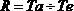where R is ratio, Ta is the number of teeth on the axle sprocket and Te is the number of teeth on the engine clutch sprocket.  We get the ratio (R) of the drive system by dividing the number of axle sprocket teeth by the number of teeth on the engine’s clutch sprocket.  Then after we know the ratio we can find the RPMw by dividing the maximum engine rpm (RPMe) by the ratio.  We get RPMw by using the following formula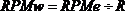.

Now we know the formulas to use and the steps to use, so let’s substitute the information we got from the kart to find how far the drive wheel moves the kart in one minute.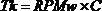.  For this formula we know C, but we don’t know RPMw yet..  For this formula we know RPMe, but we don’t know R, the ratio of the drive system yet., is the formula for ratio.  We can work this formula because we counted 60 axle teeth and 10 engine clutch teeth, so let’s substitute that information to find the ratio.  We have 60 axle teeth divided by 10 engine clutch teeth and the result is that R is equal to 6.  Now that we know the ratio we can find RPMw., substituting, we have 3000 maximum engine rpm divided by 6 which equal 500 drive wheel rotations.  Finally, we can find the distance the drive wheel moves the kart in one minute with the formula.  Tk is the distance the drive wheel moves the kart in one minute, RPMw is the number of times the drive wheel revolves in one minute and C is the distance the drive wheel moves the kart in one revolution.  Substituting, we have 500 times 3.927 feet which gives Tk equals 1963.5 ft. per minute of kart travel.

In step 3 we’ll use the last formula which is one to change feet per minute into miles per hour.  If we divide the number of feet per minute (1963.5) by the number of feet in a mile (5280) we will know the portion of a mile that the kart travels in one minute.   Then if we multiply that by the number of minutes in an hour we’ll find how far the kart will travel in an hour.  So,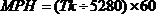is one formula we can use.  Substituting, we have 1963.5 divided by 5280 feet, which equals 0.371875 of a mile in one minute.  That multiplied by the 60 minutes in an hour is equal to 22.3125 MPH.

The MPH formula can be stated in at least two more ways and still give the correct results.  Notice the parenthesis, which tell the one solving the equation to do the operation inside the parenthesis first.  The formula can be stated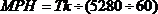and, honoring the parenthesis, the answer will be the same.  The formula can be condensed even further to.  The answer will still be correct.  If I worked the formula as stated the last way I might have gotten the correct answer, but I might not have understood why the formula worked.

KPH

The kph section will be shorter because it is a bit simpler and you have already been made familiar with the concept.

First count the sprocket teeth, measure the drive tyre outside diameter and measure the maximum engine rpm while riding at full speed, as mentioned above.  Also, please observe all safety precautions noted in the first paragraph.  For the figures we’ll use the same kart used in the previous example.

Step 1, the same 15 inch diameter drive tyre measures 381mm in the metric system.  The formula used to find the distance the drive tyre moves the kart in one rotation is.  Substituting, we have 3.1416 multiplied by 381 gives C equal to 1196.9496 Mm.  We need figures stated in Km.  You can work the conversion at any location that gives Mm by dividing Mm by 1000000 because there are 1000000 Mm in each Km.  So, 1196.9496 divided by 1000000 equals 0.0011969 Km.  So, C equals 0.0011969 Km

Step 2, already we know the sub steps to be performed from the previous explanation of mph.   We want to know the distance the kart will be moved by the drive tyre in one minute (Tk) and we know that we must find RPMw and R, and we know that to get the RPMw we must find the ratio first.  We’re using the same kart in this example with the same number of teeth on the engine and drive axle.  So, the formula for ratio is,and substituting, 60 divided by 10 gives R equals 6., substituting, the engine rpm 3000 divided by 6 gives RPMw equals 500.  Finally, we get the distance the drive wheel moves the kart in one minute by the formula.  Substituting, we get 500 times C (0.0011969 Km.) gives Tk equals 0.59845 Km per minute.

Step 3 is the easiest.  The formula for kph is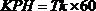.Since we already converted the distance from Mm to Km, Tk is expressed in Km here, so, we again substitute, and 0.59845 Km per minute multiplied by 60 minutes in an hour gives KPH equals 35.907 kph.

The drive system referred to, up to this point, has been the chain only type of drive.   There are two other systems that are common.  One is a belt drive system characterized by its having a belt only, which is driven by a pulley on the engine (Pe) which drives another pulley on the axle (Pa).  The ratio for this system is found by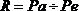, that is, dividing the measurement of the axle pulley by the measurement of the engine pulley.  The measurement of both pulleys must be the radius, the diameter or the circumference, not one measurement made of the diameter and the other measurement made of the circumference or the radius.  If it is more convenient to make measurements of pulley in diameter and the other in circumference one measurement must be converted to match the designation of the other.

Another type of drive system is the torque converter.  This system is a combination of belt and chain drive.  In research for this article I found that two torque converters were common and that the ratio for each was published.  One converter, called Torque-A-Verter 2 ™ has a ratio of about 19 initially down to about 5 at maximum speed, and the Comet ™ brand has a ratio of about 3 initially down to a ratio of 1 at maximum speed.  The torque converter with a ratio of 1 does not however make the RPMw the same as the RPMe.  For a good giggle, calculate the speed if the ratio of 1 was to the drive tire.  The 10 page version of this article has more information about finding the ratio of torque converter drive systems.

Also, in the 10 page version is information and formulas to use a combination of Method 1 and Method 2 to find your engine maximum rpm, even if you don’t have access to a tachometer.